# How to Create an Excel Pivot Table With Medians

As a marketer, you already know that Microsoft Excel is a powerful tool for sorting, analyzing, and sharing data. Trouble is, some of the most beneficial formulas are really tough to figure out — even for us data-crunchers.

For example, we've walked through the steps of how to create a pivot table before, but unfortunately pivot tables don't compute median values, which can be highly useful information with which organizations can analyze their growth.

Luckily, there is a workaround in Excel called the array, where you can use formulas to calculate the median of a data set. We'll walk you through how to do that in the example below, which features a list of customers along with their company size and sales cycle length. For context, the two tables (average vs. median) look at the typical sales cycle length for each company size.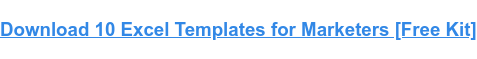## The Excel Pivot Table Alternative for Calculating Median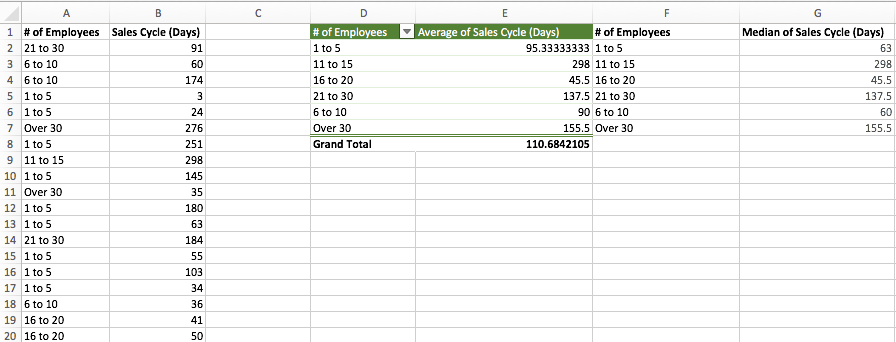The “Average of Sales Cycle (Days)” table was created with a pivot table. The “Median of Sales Cycle (Days)” table was created by doing the following:

#### 1) Create a column with the six possible “employees” options: 1 to 5, 6 to 10, 11 to 15, etc.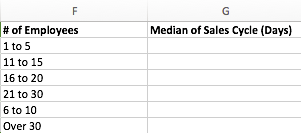###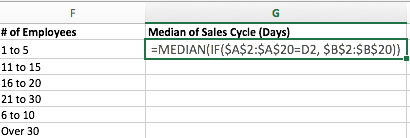#### 3) When you've closed the final parenthesis and while you're still in the cell, type Control+Shift+Enter (on a PC) or Command+Shift+Enter (on a Mac) to populate the median. This is how you tell Excel that you want to create an array.

Note: Once you do this, you will see curly brackets { } appear around your formula. If you type in the curly brackets yourself or copy/paste the formula above into the cell, Excel won't understand what they mean.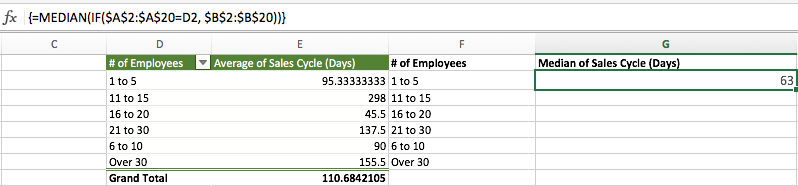#### 4) Copy the median amount in the first cell (G2 in the example) into the rest of the empty cells in the table (G3-G7 in the example).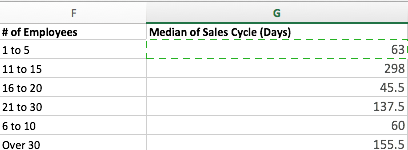Here is a breakdown of what these formula inputs mean: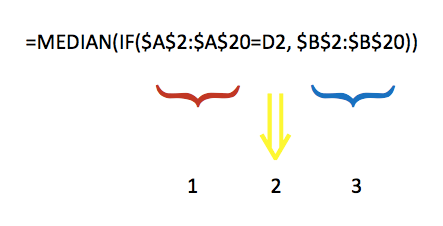1. This is the column of values for “# of Employees” in Column A
2. This is the contents of cell D2: “1 to 5”
3. This is the column of values for “Sales Cycle (Days)” in Column B

Translation: First, the IF statement finds all rows where the # of Employees = “1 to 5”; it then stores all of the corresponding “sales cycle” values in an array. The MEDIAN function then pulls the median out of that array of sales cycles for the “1 to 5” customers.

## When to Use Median to Analyze Data

Average (or mean) and median are common measures of data, but generally speaking average is more commonly used than median.

While average is a helpful way to determine what a typical member of a data set looks like, in some cases, median actually provides a fuller picture of a data set within its context.

In fact, average can actually be misleading if the data set is highly skewed and a large set of the population is similar with several far-away outliers. In such cases, the median is a better indicator of what a typical member of the data set looks like.

For example, in the United States, household income is commonly measured and referred to in terms of the median. This could be due to the fact that high-income inequality nationwide would make the average a poor representation of a typical American household's income.

Here's another scenario: If a business were analyzing sales, it would be important for them to consider the types of data in the sets they were analyzing. For example, calculating the average amount of total monthly sales would be a good indicator of performance because each month has roughly the same number of days and opportunities to sell, so the average would show what a baseline expectation of productivity would be.

However, the average may not be the best measure to analyze each sale's size that year. If the organization sold products varying in price from \$10 to \$25,000, then the average sale size might be skewed by the lowest and highest prices. Instead, the median would give the organization an idea of if sales were typically higher or lower in price. See the difference?

Ultimately, analyzing both the average and the median will provide the most full picture possible of your organization's raw data, and pivot tables in Excel, such as the ones above, can help you analyze both data sets.

Want more Excel tips? Check out this list of Excel shortcuts.This site uses Akismet to reduce spam. Learn how your comment data is processed.